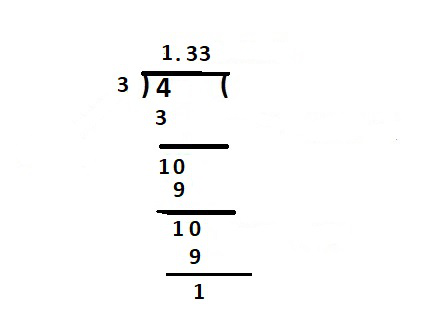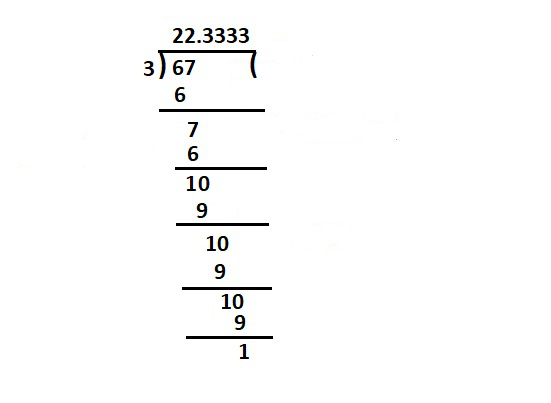# How to write a rational number as a repeating decimal?

• Last Updated : 11 May, 2022

There are different sorts of numbers that are included in the number system, such as prime numbers, odd numbers, even numbers, rational numbers, whole numbers, and so on. These numbers can be expressed in a variety of ways, including figures and words.  For example, in the form of figures, the numbers 50 and 75 can alternatively be written as fifty and seventy-five.

A number system, often known as a numeral system, is a basic system for expressing numbers and figures. It is the only way to represent numbers in both arithmetic and algebraic structures. Numbers are utilized in a variety of arithmetic values that may be used to perform a variety of arithmetic operations such as addition, subtraction, multiplication, and other operations that are used in daily life for the purpose of computation.

In the numeral system, Real numbers are simply a combination of rational and irrational numerals. All known arithmetic functions may be performed on these numbers and represented on the number line. The digit, its place value in the number and the number system’s base determine the value of a number.  The mathematical values used for counting, measuring, identifying, and measuring fundamental quantities are known as numbers or numerals.

### Rational numbers

A rational number is the one that can be written as a ratio of two integers p and q, where q is not zero. and Repeating decimals are those that have a set of decimal terms that are repeated consistently.  Different sorts of rational numbers occur. We shouldn’t assume that only rational numbers are fractions with integers. Numbers that are rational can also be stated in decimal form.

Example: Is 1.1 a rational number?

Yes, it’s a rational number because 1.1 may be represented as 1.1 = 11/10. Let’s take a look at non-terminating decimals like 0.333… 0.333… is a rational number since it may be expressed as 1/3. Non-terminating decimals with repeated numbers after the decimal point are thus rational numbers as well.

Examples of Rational numbers are,

• All numerals are rational, 1, 2, 3, 4, 5, 6, 7… all are rational numbers.
• All Whole numerals are rational. 0, 1, 2, 3, 4, 5,… all are rational.

The following are the several sorts of rational numbers,

Integers such as -2, 0, 3, and so on. Fractions with integer numerators and denominators, such as 3/7, -6/5, and so on. 0.35, 0.7116, 0.9768, and so on are examples of terminating decimals. Non-terminating decimals having recurring patterns (after the decimal point), such as 0.333…, 0.141414…, and so on.

Examples of Repeated decimals are,

6/4 as a decimal is 6 ÷ 4 = 1.5  terminating decimal. 35/100 as a decimal is 35 ÷100 = 0.35 terminating decimal. 5/3 as a decimal is 5 ÷ 3 = 1.66666… its a repeating decimal of Rational number.

### How to write a rational number as a repeating decimal?

Any rational number (a fraction in lower times) can be expressed as a terminating decimal or a repeating decimal. Simply divide the numerator by the denominator.

If there is a remainder of zero, you have a terminating decimal. Otherwise, the remainder will begin to repeat after some significance, resulting in a repeating decimal.

Example: Write rational number 4/3 in repeating decimal form.

Simply divide the numerator by the denominator.

So, here we divide 4 by 3,Repeating decimal

Here after simplifying we get 4/3 = 1.333333…

Hence, this way we can write rational number as repeating decimal.

### Similar Questions

Question 1: Write the rational number 67/3 as a repeating decimal?

Solution:

Any rational number (a fraction in lower times) can be expressed as a terminating decimal or a repeating decimal. Simply divide the numerator by the denominator. Here divide 67 by 3,67/3 as repeating decimal

We can write rational number 67/3 as a 22.33333… as repeating decimal.

Question 2: Write 11/3 rational numbers as repeating decimal?

Solution:

Any rational number (A fraction in lower times) can be expressed as a terminating decimal or a repeating decimal. Simply divide the numerator by the denominator.

Here divide 11 by 3: we can write 11 /3 as 3.666666666… after dividing,

Therefore it’s a repeating decimal of rational number.

Question 3: Write 103/6 rational number as repeating decimal?

Solution:

Any rational number (A fraction in lower times) can be expressed as a terminating decimal or a repeating decimal. Simply divide the numerator by the denominator.

Here divide 103 by 6: we can write 103 / 6 as 17.166666666… after dividing,

Therefore it’s a way of rational number writing as repeating decimal.

Question 4: Write 5/6 rational number as a repeating decimal?

Solution:

Any rational number (A fraction in lower times) can be expressed as a terminating decimal or a repeating decimal. Simply divide the numerator by the denominator.

Here divide 5 by 6: we can write 5 / 6 as 0.8333333333… after dividing,

Therefore it’s a way of rational number writing as repeating decimal.

Question 5: Write 19/3 rational numbers as repeating decimal?

Solution:

Any rational number (A fraction in lower times) can be expressed as a terminating decimal or a repeating decimal. Simply divide the numerator by the denominator.

Here divide 19 by 3: we can write 19/ 3 as 6.3333333… after dividing,

Therefore in this way 19/3 rational number can be written as a repeating decimal.

Question 6: Write 185/6 rational number as repeating decimal?

Solution:

Any rational number (A fraction in lower times) can be expressed as a terminating decimal or a repeating decimal. Simply divide the numerator by the denominator.

Here divide 185 by 6: we can write 185/ 6 as 30.8333333… after dividing,

Therefore in this way 185/6 rational number write as repeating decimal.

My Personal Notes arrow_drop_up
Recommended Articles
Page :